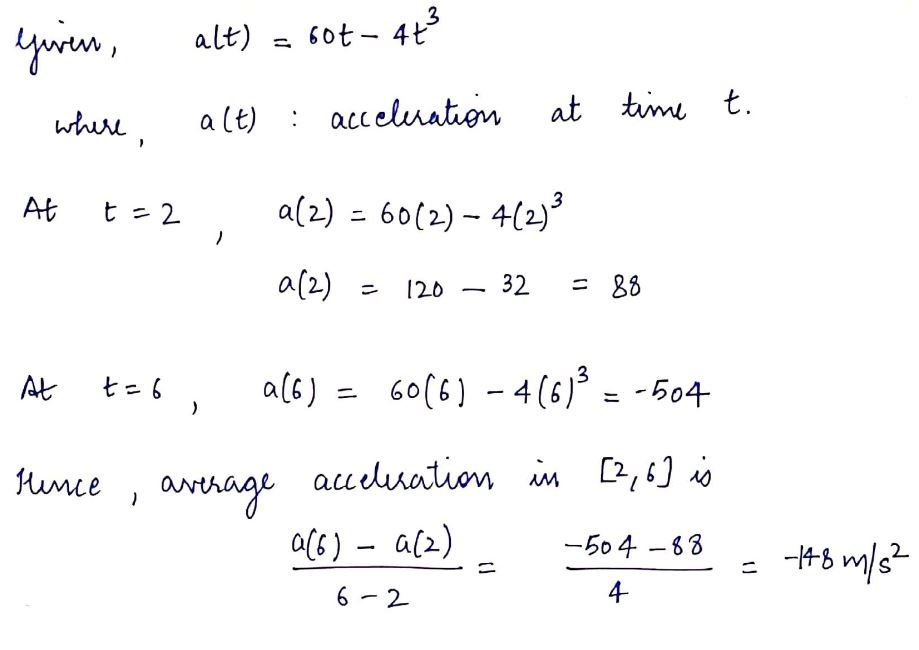# The acceleration of a particle is a(t) = 60t −4t3 m/s2. Compute the average acceleration and the average speed over the time interval [2, 6], assuming that the particle’s initial velocity is zero.

Question
14 views

The acceleration of a particle is a(t) = 60t −4t3 m/s2. Compute the average acceleration and the average
speed over the time interval [2, 6], assuming that the particle’s initial velocity is zero.

check_circle

Step 1...

### Want to see the full answer?

See Solution

#### Want to see this answer and more?

Solutions are written by subject experts who are available 24/7. Questions are typically answered within 1 hour.*

See Solution
*Response times may vary by subject and question.
Tagged in